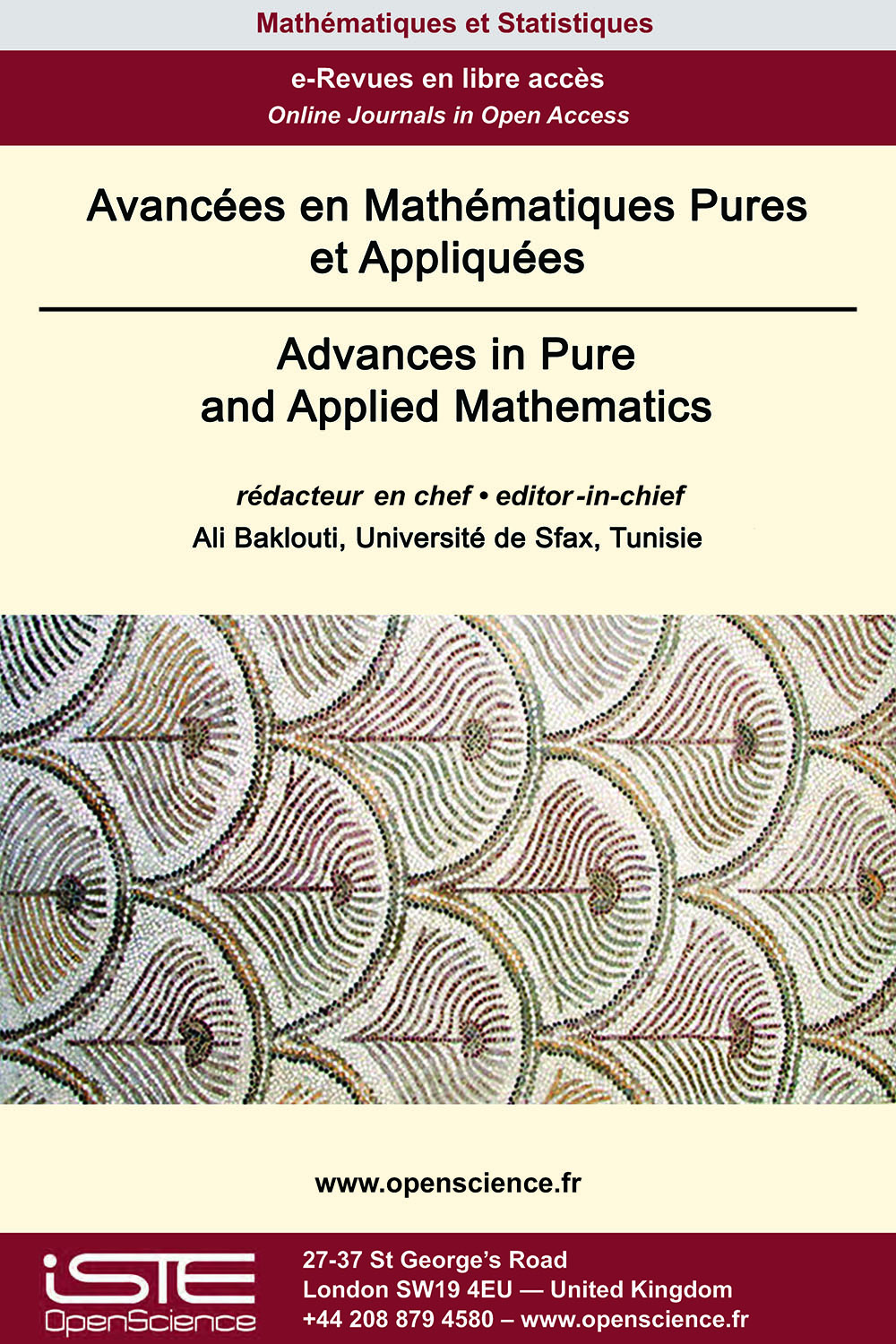# Vol 13 - Numéro 2 (Mars 2022)

## Articles parus

Formules explicites du noyau de la chaleur sur les espaces projectifs des quaternions

We consider the heat equation on the quaternionic projective space $P_{n}(ℍ)$, and we establish two formulas for the heat kernel, a series expansion involving Jacobi polynomials, and an integral representation involving a $\theta$-function. More precisely, using the quaternonic Hopf fibration and the explicit integral representation of the heat kernel on the complex projective space $P_{2n+1}(ℂ)$, as well as an integral representation of Jacobi polynomials in terms of Gegenbauer polynomials, we give an explicit integral representation of the heat kernel on the $n$-quaternionic projective space. We also establish an explicit series expansion of the heat kernel in terms of the Jacobi polynomials. Moreover, we derive an explicit formula of the heat kernel on the sphere $S^4$.

Formulation faible du laplacien sur l’espace Shift complet

We consider a Laplacian on the one-sided full shift space over a finite symbol set, which is constructed as a renormalized limit of finite difference operators. We propose a weak de-nition of this Laplacian, analogous to the one in calculus, by choosing test functions as those which have finite energy and vanish on various boundary sets. In the abstract setting of the shift space, the boundary sets are chosen to be the sets on which the finite difference operators are defined. We then define the Neumann derivative of functions on these boundary sets and establish a relation between three important concepts in analysis so far, namely, the Laplacian, the bilinear energy form and the Neumann derivative of a function. As a result, we obtain the Gauss-Green’s formula analogous to the one in classical case. We conclude this paper by providing a sufficient condition for the Neumann boundary value problem on the shift space.

Opérateurs de composition pondérés d’espaces de suites à poids

We give simple criteria which characterize the symbols $u$, $\varphi$ defining continuous and compact weighted composition operators $W_{u,\varphi}$ acting between two different weighted sequence spaces. Also we characterize when $W_{u,\varphi}$ is bounded below and when it has closed range.

Attracteurs et chaîne de récurrence pour le semi-groupe des fonctions continues

In , we extended various notions of recurrence for the action of a semigroup analogous to their counterpart in the classical theory of dynamics. In this paper, we shall address the alternative de-nition of chain recurrent set in terms of attractors, given by Hurley in  following Conley’s characterization in . We shall also discuss the notion of topological transitivity and chain transitivity in this general setting.### Autres numéros :

2020

Volume 20- 11

Numéro 1 (Mai 2020)
Numéro 2 (Septembre 2020)

2021

Volume 21- 12

Numéro 1 (Janvier 2021)
Numéro 2 (Mai 2021)
Numéro spécial : AUS-ICMS 2020
Numéro 3 (Septembre 2021)

2022

Volume 22- 13

À paraître

Numéro 1 (Janvier 2022)
Numéro 2 (Mars 2022)
Numéro 3 (Juin 2022)